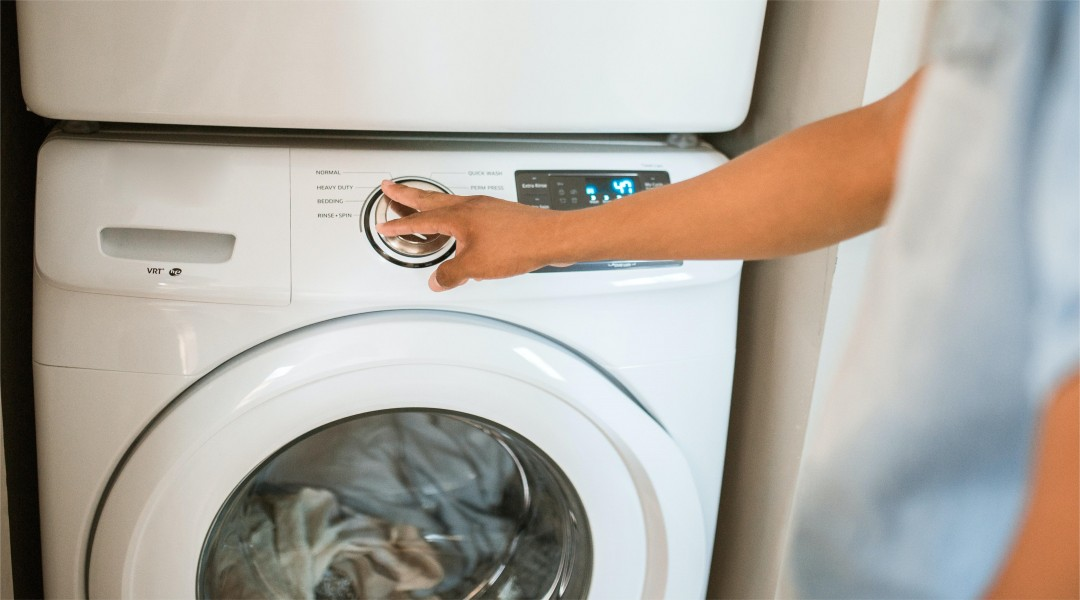# The difference between the starting watts and running watts

When you face the home power outrage or other emergency situation, Which part need to consider first when you decide to buy a portable power station. Before prepare the back-up power device, you need to have a basic understanding of the starting watts and running watts. This article will list the difference between the starting watts and running watts.

## 1.Definition

Starting watts means that the power of running device from generator, it also called surged watts, which mean the instantaneous power boost a generator can provide for starting a motor, and it need massive electricity to turn within a short time when the electric motor start up. The electric motor can reach a high speed as the increase speed and the decrease current, the power of electric motor in a stable stage. Running watts means the generator can provide power all the times, also known as the rated watts or continuous watts.## 2.Importance of Starting watts

If not have the relevant knowledge of surge and start watts, it will cause the device, the generator to be damage, and in the worst case, it can cause a fire. Starting watts is amount of wattage required to get a device running and is the work required over time to create the power needed to run the device. The generator can temporarily supply that wattage in brief surges. If the starting power requirement over the output capacity of generator, you will hard to open it. The watts requirement of running and starting devices is different. Most device not need surge watts can start up, some need more than twice the work power can be start up. Need surge power only few seconds before the unit starts operating at its operating wattage rating.

## 3.Importance of Running Watts

Running watts is the amount of power consumed continuously by a device. All electronic device need to consume the basic electricity not to be closed. The electricity is provided by the constant flow of electrons from the power source to the device, The electrons will be disrupted when you turn aff the switch and home appliance accordingly be closed. The basic amounts of the continuous energy the device demanded that is the running watts, you can take the running watts as the consume energy in maintenance mode.## 4.Calculate the starting watts and running watts

These two watts both in watts or kilowatts, Some manufacturer may aim the maximum power of generator is 3000 watts and the rated continous power is 2400 watts, So how to calculate the starting watts and running watts?

As we all know, the formula for calculating wattage for a device is: Volts (V) x Amps (A) = Watts (W),

You should change the V/A to the W. The running watts is the add the running wattages of the appliances you will use together, and check if the total running watts exceed the running watts listed on the generator. If exceed, you need to buy the massive output capacity one.

Generally, the starting watts is 2-5 times higher than running watts, If some electronic device remarked LRA at the back， you only need to multiply LRA by volts and then you get the starting watts. But not all the device had remarked LRA at the outside wrap, you can check the user manual or use the wattmeter to measure.

## 5.Conclusion

When choose the generator for what purpose, especially when the electric motor forms part of the total electrical load supplied by the generator, you need two concern these two watts of generator and figure out the difference between them.